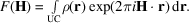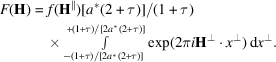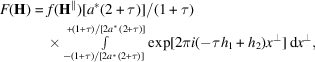International
Tables for
Crystallography
Volume B
Reciprocal space
Edited by U. Shmueli

International Tables for Crystallography (2010). Vol. B, ch. 4.6, pp. 604-605   | 1 | 2 |

## Section 4.6.3.3.1.3. Structure factor

W. Steurera* and T. Haibacha

aLaboratory of Crystallography, Department of Materials, ETH Hönggerberg, HCI G 511, Wolfgang-Pauli-Strasse 10, CH-8093 Zurich, Switzerland
Correspondence e-mail:  walter.steurer@mat.ethz.ch

#### 4.6.3.3.1.3. Structure factor

| top | pdf |

The structure factor of a periodic structure is defined as the Fourier transform of the density distributionof its unit cell (UC):The same is valid in the case of the nD description of a quasiperiodic structure. The parallel- and perpendicular-space components are orthogonal to each other and can be separated. In the case of the 1D Fibonacci sequence, the Fourier transform of the parallel-space component of the electron-density distribution of a single atom gives the usual atomic scattering factor. Parallel to,adopts valuesonly within the intervaland one obtainsThe factorresults from the normalization of the structure factors to. Withandthe integrand can be rewritten asyieldingUsinggivesThus, the structure factor has the form of the functionwith x a perpendicular reciprocal-space coordinate. The upper and lower limiting curves of this function are given by the hyperbolae(Fig. 4.6.3.6). The continuous shape ofas a function ofallows the estimation of an overall temperature factor and atomic scattering factor for reflection-data normalization (compare Figs. 4.6.3.6and 4.6.3.7).Figure 4.6.3.7 | top | pdf |The structure factorsof the Fibonacci chain decorated with aluminium atoms (Uoverall = 0.005 Å2) as a function of the parallel (above) and the perpendicular (below) component of the diffraction vector. The short distance is, all structure factors withinhave been calculated and normalized to.

In the case of a 3D crystal structure which is quasiperiodic in one direction, the structure factor can be written in the formThe sum runs over all n averaged hyperatoms in the 4D unit cell of the structure. The geometric form factorcorresponds to the Fourier transform of the kth atomic surface,normalized to, the area of the 2D unit cell projected upon, and, the area of the kth atomic surface.

The atomic temperature factorcan also have perpendicular-space components. Assuming only harmonic (static or dynamic) displacements in parallel and perpendicular space one obtains, in analogy to the usual expression (Willis & Pryor, 1975),withThe elements of the typerepresent the average values of the atomic displacements along the ith axis times the displacement along the jth axis on the V basis.

### References

Willis, B. T. M. & Pryor, A. W. (1975). Thermal Vibrations in Crystallography. Cambridge University Press.